• 自己的简单的c++小项目，学生信息管理系统，很多代码均已经附上注释，足以应付课设以及答辩，大二时亲测
• 开发一个学生信息管理系统，学生信息类中包含四个成员：学号、姓名、数学成绩、语文成绩。 系统可以实现以下功能： 添加学生信息、删除学生信息、修改学生信息、查找学生信息、列表显示学生信息、按成绩排序信息、...
• 学生信息管理系统，包括管理员和学生两大模块，管理员具有班级管理，教师管理，学生管理，课程管理，学生选课，成绩管理六个模块功能，学生具有修改密码，课程浏览，选课浏览，个人选课，成绩查询，个人信息六个模块...
• 实现功能输入输出插入删除查找追加读入显示保存拷贝排序索引分类合计退出 能实现对学生信息的简单管理 具体要求 建立一个4个学生的信息登记表每个学生的信息包括学号姓名和3门课程的成绩FOX,C,ENGLISH ?程序运行时...
• cout请输入学生（学号、姓名、成绩）;"; cin>>x.no>>x.name>>x.score; for(j=last;j>=i-1;j--) { data[j+1]=data[j]; } data[i-1]=x; last++; cout插入后信息："; print_seqlist(); return(1); } int aclass:...
#include  #include #include<string.h>
using namespace std; #define MAXSIZE 20
class aclass; class student { private: long no; char name; float score; public: student(); friend class aclass;//友元内的定义，aclass 中的成员函数均有资格访问student的数据成员，反之不可。
}; class aclass { private: char cname; student data[MAXSIZE]; int last; public: aclass(); int insert_seqlist(int i,student x); int delete_seqlist(int i); void print_seqlist();
};
int main() { aclass sq; int n,m=1; while(m) { menu(); cin>>n; switch(n) { case 1: { int i; student x; cout<<“请输入位置”<<endl; cin>>i; sq.insert_seqlist(i,x);
            break;
}
case 2:
{
int i;
cout<<"请输入删除的位置：";
cin>>i;
sq.delete_seqlist(i);
cout<<endl<<"删除后信息:"<<endl<<endl;
break;

}

case 0:m=0;
}

}
return 0;

}
void menu() { cout<<endl<<“1.插入”<<endl; cout<<“2.删除”<<endl; cout<<“0.退出”<<endl; cout<<endl<<“请选择:”;
}
student::student() { no=0; strcpy(name,""); score=0;
}
aclass::aclass() { cout<<“请输入班级名称”<<endl; cin>>cname; last=-1; }
int aclass::insert_seqlist(int i,student x) {
int j;
if(last==MAXSIZE-1)
{
cout<<"table is full!"<<endl;
return(-1);
}
if(i<1||i>(last+2))
{
cout<<"位置错误"<<endl;
return(0);
}

cout<<"请输入学生（学号、姓名、成绩）;"<<endl;
cin>>x.no>>x.name>>x.score;

for(j=last;j>=i-1;j--)
{
data[j+1]=data[j];
}
data[i-1]=x;
last++;
cout<<endl<<"插入后信息："<<endl<<endl;
print_seqlist();
return(1);

}
int aclass::delete_seqlist(int i) { int j; if(i<1||i>(last+1)) { cout<<“位置错误！”<<endl; return(0); } for(j=i;j<=last;j++) { data[j-1]=data[j]; } last–; return(1);
} void aclass::print_seqlist() { int i; cout<<“班级”<<cname<<endl; cout<<“学生”<<endl; for(i=0;i<=last;i++) { cout<<data[i].no<<setw(8)<<data[i].name<<setw(4)<<data[i].score<<endl; } cout<<endl; }
展开全文• XX大学 二一二二一三年第二学期 xxxxxxx学院 面向对象C++语言课程设计汇报 课程名称 面向对象C++语言课程设计 班 级 _ 学 号 _ 姓 名 _ 指导老师_ _ 二一三年 六 月 目录 TOC \o "1-3" \h \z \u 一系统需求和功效...
• 该软件可以对学生信息如学号,成绩等进行查找，删除，增加等。
• 1.录入学生信息：用户可以自由输入学生信息系统中。 2.打印学生信息：格式化展示系统中的学生信息。 3.保存学生信息：将系统中的学生信息保存到本地文档。 4.读取学生信息：读取本地文档中的学生信息并显示。 5....
• 带界面的学生信息管理系统/学生选课系统，增删改查等功能俱全，包含教师端和学生端，学生可按照学分进行选课，教师可对学生的信息进行管理。
• 学生信息管理系统，使用C++，亲测可用，这个参考别人的代码，但是修改了好多，本来还不能用，修改后可以使用。适合C++小白。 使用前须在D盘下新建一个student.txt 或者你自己改路径。我使用CodeBlocks运行的。
• ## C++学生成绩管理系统

万次阅读 多人点赞 2019-10-04 20:26:09
c++实现学生成绩管理系统，此系统可以提供成绩的录入，可以按学生成绩进行排名，求总分和平均分以及提供成绩查询功能等。 实现功能 提供成绩录入 统计每个学生的总分和平均分 按总分由大到小排出名次 提供成绩...
用c++实现学生成绩管理系统，此系统可以提供成绩的录入，可以按学生成绩进行排名，求总分和平均分以及提供成绩查询功能等。
实现功能
提供成绩录入统计每个学生的总分和平均分按总分由大到小排出名次提供成绩查询，就是输入一个学号，查出该学生的成绩信息
系统的实现
代码主要分为三个部分，一部分是结构和变量定义部分，另一部分是主函数部分，最后一部分是功能函数部分。具体代码如下：
结构和变量定义部分：
//结构和变量定义部分
#include "pch.h"
#include"out.h"
#include <iostream>
#include<fstream>
#include <string>
#include<iomanip>
#include <fstream>
#include<strstream>
#include <vector>
#include<cstdio>

using   namespace   std;
const int Max = 30;		//学号和字符串的大小
//函数的声明
class Student;
void setData(Student &s);
void count(Student &s);
void sort(Student S[], int N);
double getAverage(Student S[], int N);
void print(Student &s);
int search(Student S[], int N, char *n);
class Student
{
public:		//定义类的公有成员
char number[Max];	//学号
char name[Max];		//姓名
double chinese;		//语文
double math;		//数学
double english;		//英语
double total;		//总分
double average;		//平均分
int rank;			//排名
};

主函数部分：
//主函数部分
int main()
{
const int M = 3;	//定义常量，假设为3位同学
Student S[M];		//创建对象数组
for (int i = 0; i < M; i++)
{
cout << "输入下面第" << i + 1 << "位同学的数据：" << endl;
setData(S[i]);		//调用数据输入函数
count(S[i]);
cout << endl;
}
sort(S, M);
int order = 1;
while (order != 4)
{
cout << "****************************命令菜单****************************" << endl;
cout << "1.查询所有排名" << endl;
cout << "2.查询成绩在全班平均分以上的学生名单和信息" << endl;
cout << "3,任意输入一个学号，查找该学生的排名和考试成绩" << endl;
cout << "4.退出系统" << endl;
cout << "****************************************************************" << endl;
cout << "请输入命令：";
cin >> order;
switch (order)
{
case 1:
{
for (int j = 0; j < M; j++)
print(S[j]);	//输出对象数组
}
break;
case 2:
{
double compare = getAverage(S, M);		//获取平均分，之后将大于平均分的同学输出
for (int k = 0; k < M; k++)
if (S[k].average > compare)
print(S[k]);
}
break;
case 3:
{
char code[Max];
cout << "输入您要查找的学号：";
cin >> code;
int result = search(S, M, code);
if (result == -1)
cout << "您输入的学号不存在！" << endl;
else
print(S[result]);
}
break;
case 4:
break;
default:
cout << "输入错误，请重新输入" << endl;
}
}
}

功能函数部分：
//功能函数部分
void setData(Student &s)
{
cout << "输入学号，姓名，语文，数学，英语成绩：";
cin >> s.number >> s.name >> s.chinese >> s.math >> s.english;		//分别将输入的数据赋给类的成员
//成员的初始化
s.total = 0;
s.average = 0;
s.rank = 0;
}

void count(Student &s)
{
s.total = s.chinese + s.math + s.english;		//求总分
s.average = s.total / 3;		//求平均分
}

void sort(Student S[], int N)		//插入法排序
{
int index;
Student inserter;
for (int i = 1; i < N; i++)		//依次比较所有学生
{
inserter = S[i];		//取出元素i
index = i - 1;
while (index >= 0 && inserter.average > S[index].average)
{		//该元素大于第i-1个元素
S[index + 1] = S[index];
index--;
}
S[index + 1] = inserter;		//插入
}
for (int j = 0; j < N; j++)
S[j].rank = j + 1;		//设置排名
}

double getAverage(Student S[], int N)
{
double Average = 0;
for (int i = 0; i < N; i++)
Average += (S[i].chinese + S[i].math + S[i].english);
Average /= (N * 3);
return Average;
}

void print(Student &s)
{
cout << "排名" << "\t" << "学号" << "\t" << "姓名" << "\t" << "语文" << "\t" << "数学" << "\t" << "英语"
<< "\t" << "总分" << "\t" << "平均分" << endl;
cout <<s.rank<< "\t" << s.number << "\t" << s.name << "\t" << s.chinese << "\t" <<s.math << "\t" << s.english
<< "\t" << s.total << "\t" << s.average << endl;
}

int search(Student S[], int N, char *n)
{
for (int i = 0; i < N; i++)
{
if (strcmp(S[i].number, n) == 0)		//比较字符，如果n(用户输入的学号)与系统中学号相等，strcmp(S[i].number, n)值为0
return i;		//返回下标
}
return -1;
}

将以上三个部分合起来就能运行程序了哦！
程序运行结果
输入3（3是数组的大小，可修改）位同学的数据后就能进入系统了，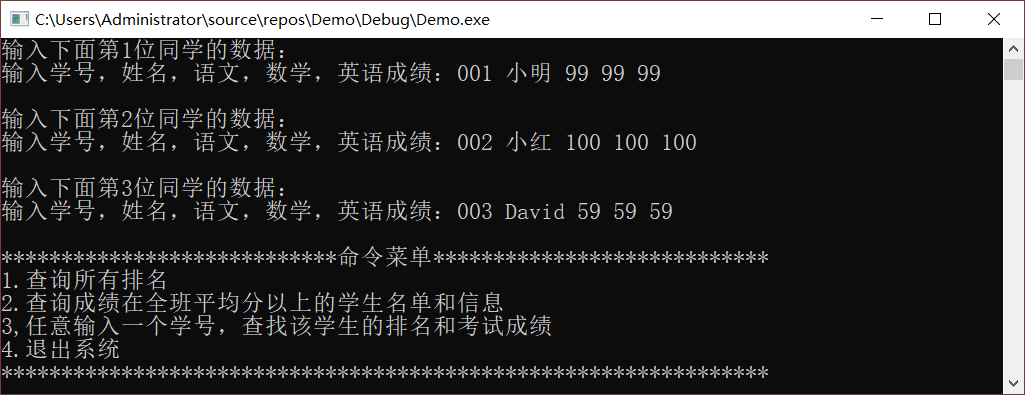查询排名情况：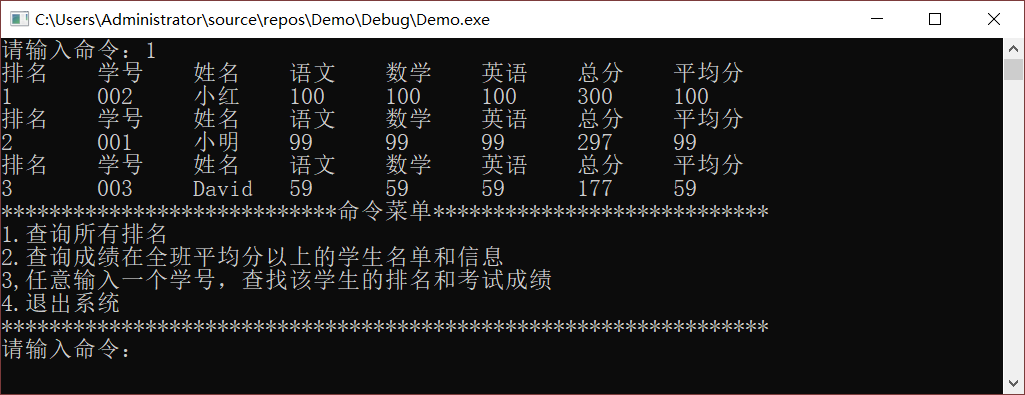查询高于平均分的同学：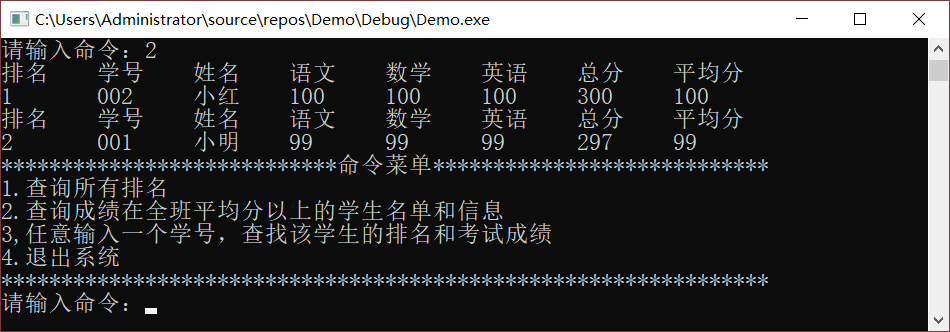输入学号查看学生信息：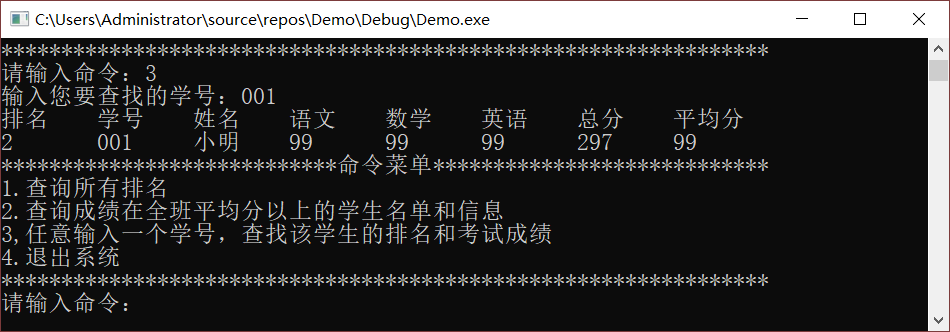最后是退出：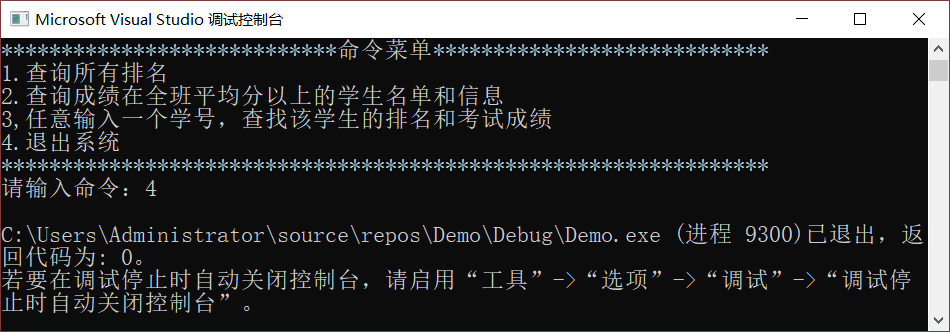功能已全部实现，虽然目前程序还很简陋，但我们可以开发更多的功能去进一步完善它。只要功夫深，铁杵磨成针，希望能帮助到有需要的小伙伴，互相学习，一起进步！展开全文• C++课程设计期末大作业，学生信息管理系统，主要实现功能：1.添加学生信息2.修改学生信息3.删除学生信息4.显示学生信息 5.保存学生信息 6.查找学生信息 0.退出
• C++实现学生信息管理系统[界面+数据处理]，是个人博客中需要的工程文件，主要实现界面化，信息录入，信息查找，信息删除，信息浏览的功能
• 需要提前下载MySQL数据库，该工程基于Qt5完成图形化界面设计，有登录界面，可以选择管理员、学生两种身份登录，对应不同的操作权限。
• c++和数据库Sqlite实现一个“学生信息管理”软件 (1) 增加 (2) 删除 (3) 列表显示 (4) 查询（按名称匹配） (5) 数据存盘（永久化存储） (6) 设置与显示头像 (一寸照片, JPG)
• 这是本人做的一个基于DOS窗口的学生管理系统，使用的是VS2012的平台，该系统经过海量的数据测试，基本把所有的bug都给排除掉了，该系统可以提供给初学者进行学习使用。
• 老师，学生，选课 用二叉树查找，百万信息秒查 老师，学生，选课 用二叉树查找，百万信息秒查 老师，学生，选课 用二叉树查找，百万信息秒查 老师，学生，选课 用二叉树查找，百万信息秒查
• ## 基于C++的学生信息管理系统的实现

千次阅读 多人点赞 2020-07-03 09:37:01
在完成一学期的C++语言学习之后，一个学生信息管理系统来丰富自己的实战经验。 课设内容的要求有主菜单模块、输入模块、查询模块、更新模块、排序模块、统计模块和输出模块，并且通过主菜单模块调用各个子模块。 ...
在完成一学期的C++语言学习之后，写一个学生信息管理系统来丰富自己的实战经验。
课设内容的要求有主菜单模块、输入模块、查询模块、更新模块、排序模块、统计模块和输出模块，并且通过主菜单模块调用各个子模块。 主菜单模块须有循环嵌套语句实现用户不断对功能进行选择，并实现首次使用系统有录入信息的功能。 输入模块需要通过键盘输入信息并能输出到文件，说明程序必须包括文件操作流。 查询模块可分为两个子模块，分别通过姓名查询和学号查询并显示相应查询结果。 更新模块不仅更新还要确认是否保存到文件，要有if语句的运用。这样就可以结合输入模块运用同一个函数确认是否保存到文件。 排序模块可选择冒泡排序或者选择排序法，也需要将排序结果输出到屏幕，此时可以与其他有关的需要输出到屏幕上的模块调用同一个输出函数。 统计模块用if语句实现对各个区间的分数段实现输出到屏幕。 输出模块需要对所有信息进行输出，主要在排版问题上，要注意排版问题。用setw控制长度，在主函数里用switch（）-case来控制用户的选择功能，调用相应函数，应用清屏函数相应美化界面会使用户获得较好的系统使用体验值。
源码如下所示
#include<iostream>
#include<fstream>
#include<conio.h>        //调用getch()的头文件
#include<string.h>       //对字符串的操作函数调用的头文件
#include<iomanip>
#include<stdlib.h>       //调节颜色头文件
using namespace std;
struct student
{
char number;
char name;
char sex;
double score;
}Stu;
void input();             //输入学生信息
void save();              //保存学生信息
void search();            //查找学生信息
void search_number();     //按学号查找
void search_name();       //按姓名查找
void print(int i);	      //单个输出函数
void output();            //输出学生信息
void clean();             //清屏
void del();               //删除学生信息
void change();            //修改学生信息
void order();             //排序
void Norder(int type);    //排序小函数
void no();                //统计各科各分数段以及不及格人数
int N=0;
int main()
{
system("color F0");
cout<<"                                            欢迎来到学生成绩管理系统!!!\n"<<endl;
{
cout<<"                                      --请输入任意键继续,进入个人信息页面--\n"<<endl;
getch();

//以下为输入学生信息模块
system("color 1E");
cout << "文件已经录入了" << N << "组数据,若仍需要增加,请您在管理系统中使用添加功能继续添加:\n";
cout << "--请按任意键继续--\n";
getch();
system("CLS");
//以下为管理功能模块
int n;//n为功能选择参数
while (true)
{
system("color 1E");
cout <<"                                           欢迎来到学生管理系统!!!\n"<<endl;
cout << "                                             共有" << N << "名学生" << endl;
cout << "                                            请选择所需要的功能0-8" << endl;
cout << "                                            1-对学生信息进行输入" << endl;
cout << "                                            2-对学生信息进行查看" << endl;
cout << "                                            3-对单一学生信息进行查找" << endl;
cout << "                                            4-对学生信息进行删除" << endl;
cout << "                                            5-对学生信息进行修改" << endl;
cout << "                                            6-对学生信息进行增添" << endl;
cout << "                                            7-对学生信息进行排序输出" << endl;
cout << "                                            8-对学生信息进行统计" << endl;
cout << "                                            0-退出" << endl;
cin >> n;
system("cls");
switch (n)
{
case 1:
{
input();
clean();
break;
}
case 2:
{

output();
clean();
break;
}
case 3:
{
search();
break;
}

case 4:
{
del();
clean();
break;
}
case 5:
{
change();
clean();
break;
}
case 6:
{
clean();
break;
}
case 7:
{
order();
clean();
break;
}
case 8:
{
no();
clean();
break;
}
case 0:
{
system("cls");
cout<<"****************************************************"<<endl;
cout<<"————老师您辛苦了，谢谢老师！————"<<endl;
cout<<"****************************************************"<<endl;
return 0;
}
default:
{
system("color FB");
cout<<"输入错误，请重新输入!\n"<<endl;
system("CLS");
break;
}
}
}
}

return 0;
}

//输入学生信息
void input()
{
system("color 20");
cout<<"-------------输入学生信息-----------"<<endl;
int n;
cout << "首次输入学生信息个数为" << endl;
cin >> n;
for(int i=0;i<n;i++)
{
cout<<"-------------输入学生信息-----------"<<endl;
cout<<"请输入学生的学号:"<<endl;
cin>>Stu[i].number;
cout<<"请输入学生的姓名:"<<endl;
cin>>Stu[i].name;
cout<<"请输入学生的性别:"<<endl;
cin>>Stu[i].sex;
cout<<"请输入学生的高等数学成绩:"<<endl;
cin>>Stu[i].score;
cout<<"请输入学生的英语成绩:"<<endl;
cin>>Stu[i].score;
cout<<"请输入学生的计算机成绩:"<<endl;
cin>>Stu[i].score;
Stu[i].score = (Stu[i].score + Stu[i].score + Stu[i].score) / 3;
N++;
save();
system("cls");
}
}

//读取学生信息
{
fstream fcin;
fcin.open("zxl.txt",ios::in);
N=0;
for(int i=0;!fcin.eof();i++)//eof是文件末尾，文件没读到文件末尾
{
fcin>>Stu[i].number;
fcin>>Stu[i].name;
fcin>>Stu[i].sex;
fcin>>Stu[i].score;
fcin>>Stu[i].score;
fcin>>Stu[i].score;
Stu[i].score=(Stu[i].score+Stu[i].score+Stu[i].score)/3;
if((Stu[i].number>='0')&&(Stu[i].number<='9'))
N++;
}
fcin.close();
}

//输出学生信息
void output()
{
int i;
system("color B4");
cout << "查看学生信息\n";
cout << left;//左对齐
cout << "---------------------------------------------长大学生登记信息表-----------------------------------\n";
cout << "|学号        |姓名         |性别         |数学        |英语       |计算机      |平均分        " << endl;
cout << "--------------------------------------------------------------------------------------------------------------\n";
for (i = 0; i<N; i++)
{
cout << "|" << setw(14) << Stu[i].number << "|" << setw(14) << Stu[i].name << "|" << setw(14) <<Stu[i].sex
<< "|" << setw(14) <<Stu[i].score << "|" << setw(14) << Stu[i].score << "|" << setw(14) << Stu[i].score << "|"
<< setw(14) <<Stu[i].score << endl;
cout << "--------------------------------------------------------------------------------------------------------------\n";
}
clean();
}

//保存学生信息
void save()
{
ofstream fcout("zxl.txt", ios::trunc);//ios::trunc是覆盖原有内容（课本236页）
int i;
for (i = 0; i<N; i++)
{
fcout <<Stu[i].number << "\t" << Stu[i].name << "\t" <<Stu[i].sex << "\t" <<Stu[i].score << "\t"
<<Stu[i].score << "\t" <<Stu[i].score <<endl;
}
fcout.close();
}
//查找学生信息
void search()
{
system("color 82");
int n;
while (true)
{
cout << "1	通过学号查找；" << endl;
cout << "2	通过姓名查找；" << endl;
cout << "0	返回上一级；" << endl;
cin >> n;
system("cls");
switch (n)
{
case 1:
{
search_number();
getch();
break;
}
case 2:
{
search_name();
getch();
break;
}
case 0:
{
return;
}
default:
{
cout << "输入错误！请重新输入" << endl;
getch();
continue;
}
}

}
}
//按学号查找
void search_number()
{
int i;
char number;
int flag = 0;
cout << "请输入学号：" << endl;
cin >> number;
for (i = 0; i < N; i++)
{
if (strcmp(Stu[i].number ,number)==0)
{
flag = 1;
print(i);
}
}
if (flag==0)
cout << "没有该生！" << endl;
}
//按姓名查找
void search_name()
{
int i;
int flag = 0;
char name;
cout << "请输入姓名" << endl;
cin >> name;
for (i = 0; i < N; i++)
{
if (strcmp( Stu[i].name , name)==0)
{
print(i);
flag = 1;
}
}
if(flag==0)
cout << "没有该生！" << endl;
}
//输出单个学生信息
void print(int i)
{
cout << "找到该生！（顺序为：学号、姓名、性别、高数成绩、英语成绩、计算机成绩、平均成绩）"<<endl;
cout << Stu[i].number << "\t" << Stu[i].name << "\t" << Stu[i].sex
<< "\t" << Stu[i].score << "\t" << Stu[i].score << "\t" <<
Stu[i].score <<"\t"<<(Stu[i].score+ Stu[i].score+ Stu[i].score)/3<<endl;
}

//添加学生信息
{
system("color 4B");
N=N+1;
cout<<"-------------添加学生信息-----------"<<endl;
cout<<"请输入添加学生的学号:"<<endl;
cin>>Stu[N-1].number;
cout<<"请输入添加学生的姓名:"<<endl;
cin>>Stu[N-1].name;
cout<<"请输入添加学生的性别:"<<endl;
cin>>Stu[N-1].sex;
cout<<"请输入添加学生的高等数学成绩:"<<endl;
cin>>Stu[N-1].score;
cout<<"请输入添加学生的英语成绩:"<<endl;
cin>>Stu[N-1].score;
cout<<"请输入添加学生的计算机成绩:"<<endl;
cin>>Stu[N-1
].score;
Stu[N - 1].score = (Stu[N - 1].score + Stu[N - 1].score + Stu[N - 1].score) / 3;
cout<<"是否保存？(1是0否)"<<endl;
int z;
cin>>z;
if(z==1)
save();
else
{
N--;
cout << "未存入原\"zxl.txt\"文件" << endl;
}
}

//删除学生信息
void del()
{
system("color 60");
int n, x;
while (true)
{
cout << "1	按学号查找并删除" << endl;
cout << "2	按姓名查找并删除" << endl;
cout << "0	返回上一级      " << endl;
cin >> n;
switch (n)
{
case 1:
{
int i;
char number;
int flag = 0;
cout << "请输入学号：" << endl;
cin >> number;
for (i = 0; i < N; i++)
{
flag = i;

if (strcmp(Stu[i].number , number)==0)
{
print(i);
for (int a = flag-1; a < N-1; a++)//flag-1是因为i++导致最后多了一个
{
Stu[a] = Stu[a + 1];
N--;
}
cout << "是否保存本次删除？(1是，0否)" << endl;
cin >> x;
if (x==1)
{
cout << "已添加到文件" << endl;
save();
}
else
{
break;
}
}
else
{
cout << "输入错误！" << endl;
break;
}
}

break;
}
case 2:
{
int i;
int flag = 0;
char name;
cout << "请输入姓名" << endl;
cin >> name;
for (i = 0; i < N; i++)
{
flag=i;
if (strcmp(Stu[i].name, name) == 0)
{
print(i);
for (int a = flag-1; a < N; a++)//flag-1是因为i++导致最后多了一个
{
Stu[a] = Stu[a + 1];
N--;
}
cout << "是否保存本次删除？(1是，0否)" << endl;
cin >> x;
if (x==1)
{
cout << "已添加到文件" << endl;
save();
}
else
{
break;
}
}
else
{
cout<<"输入错误！"<<endl;
break;
}
}

break;
}
case 0:
{
return;
}
default:
{
cout << "输入错误！请重新输入" << endl;
continue;
}
}
}
}

//修改学生信息
void change()
{
system("color 37");
int n, x, m;
while (true)
{
getch();
system("cls");
cout << "1	按学号查找并修改" << endl;
cout << "2	按姓名查找并修改" << endl;
cout << "0  返回上一级       " <<endl;
cin >> n;

switch (n)
{
case 1:
{
int i,x;
char number;
int flag = 0;
cout << "请输入学号：" << endl;
cin >> number;
for (i = 0; i < N; i++)
{
if (strcmp(Stu[i].number , number)==0)
{
flag = i;
print(i);
cout<<"修改该生的	1-高数成绩	2-英语成绩	3-计算机成绩" << endl;
cin >> m;

if (m == 1)
{
cout << "将该科成绩修改为" << endl;
cin >> Stu[flag].score;
}
else if (m == 2)
{
cout << "将该科成绩修改为" << endl;
cin >> Stu[flag].score;
}
else if (m == 3)
{
cout << "将该科成绩修改为" << endl;
cin >> Stu[flag].score;
}
else
{
cout << "输入有误！请重新输入" << endl;
continue;
}
cout << "是否确认保存修改？(1是，0否)" << endl;
cin >> x;
if (x == 1)
{
cout << "已保存修改" << endl;
save();
}
else
{
}
clean();
}
else
{
cout<<"按任意键返回"<<endl;
continue;
}
}

break;
}
case 2:
{
int i;
int flag = 0;
char name;
cout << "请输入姓名" << endl;
cin >> name;
for (i = 0; i < N; i++)
{
if (strcmp(Stu[i].name, name) == 0)
{
flag = i;
print(i);
cout << "修改该生的	1-高数成绩	2-英语成绩	3-计算机成绩" << endl;
cin >> m;

if (m == 1)
{
cout << "将该科成绩修改为" << endl;
cin >> Stu[flag].score;
}
else if (m == 2)
{
cout << "将该科成绩修改为" << endl;
cin >> Stu[flag].score;
}
else if (m == 3)
{
cout << "将该科成绩修改为" << endl;
cin >> Stu[flag].score;
}
else
{
cout << "输入有误！请重新输入" << endl;
continue;
}
cout << "是否确认保存修改？(1是，0否)" << endl;
cin >> x;
if (x == 1)
{
cout << "已保存修改" << endl;
save();
}
else
{
}
clean();
}
else
{
cout<<"按任意键返回"<<endl;
continue;
}
}

break;
}
case 0:
return;
default:
{
cout<<"输入错误，请重新输入！"<<endl;
continue;
}
}
}
}

//清屏 没错误
void clean()
{
cout << "--请按任意键返回--\n"<<endl;
getch();
system("CLS");
}

//排序 没错误
void order()
{
system("color A8");
cout<<"请输入排序方式(1-4):"<<endl;
cout<<"1————按高数成绩排序"<<endl;
cout<<"2————按英语成绩排序"<<endl;
cout<<"3————按计算机成绩排序"<<endl;
cout<<"4————按平均成绩排序"<<endl;
int x;
cin>>x;
if(x==1||x==2||x==3||x==4)
{
Norder(x-1);
output();
int b;
cout << "是否将排序结果存入文件?(1是，0否)" << endl;
cin >> b;
if (b==1)
save();
else
{
cout << "未存入原\"zxl.txt\"文件" << endl;
}
}
else
cout<<"输入错误,";
}
void Norder(int type)//排序小函数(冒泡排序）
{
int i, j;
student t;
int flag;
for (i = 0; i<N; i++)
{
flag = 0;
for (j = 0; j<N - i - 1; j++)
if (Stu[j + 1].score[type]> Stu[j].score[type])
{
t =Stu[j + 1];
Stu[j + 1] = Stu[j];
Stu[j] = t;
flag = 1;
}
if (flag == 0)break;
}
}

//统计各科各分数段以及不及格人数
void no()
{
system("cls");
cout<<"请输入统计方式（1-4）"<<endl;
cout<<"1——平均分\n"<<"2——高数\n"<<"3——英语\n"<<"4——计算机\n"<<endl;
int p;
cin>>p;
int i;
int a=0,b=0,c=0,d=0,e=0;

switch(p)
{
case 1:
{
for(i=0;i<N;i++)
{
if(Stu[i].score>=90)
a++;
else if(Stu[i].score>=80)
b++;
else if(Stu[i].score>=70)
c++;
else if(Stu[i].score>=60)
d++;
else
e++;
}
break;
}
case 2:
{
for(i=0;i<N;i++)
{
if(Stu[i].score>=90)
a++;
else if(Stu[i].score>=80)
b++;
else if(Stu[i].score>=70)
c++;
else if(Stu[i].score>=60)
d++;
else
e++;
}
break;
}
case 3:
{
for(i=0;i<N;i++)
{
if(Stu[i].score>=90)
a++;
else if(Stu[i].score>=80)
b++;
else if(Stu[i].score>=70)
c++;
else if(Stu[i].score>=60)
d++;
else
e++;
}
break;
}
case 4:
{
for(i=0;i<N;i++)
{
if(Stu[i].score>=90)
a++;
else if(Stu[i].score>=80)
b++;
else if(Stu[i].score>=70)
c++;
else if(Stu[i].score>=60)
d++;
else
e++;
}
break;
}
default:
{
cout<<"输入错误，请重新输入！"<<endl;
}
}
cout<<"该科成绩："<<endl;
cout<<"九十分以上的有"<<a<<"个"<<endl;
cout<<"八十分以上的有"<<b<<"个"<<endl;
cout<<"七十分以上的有"<<c<<"个"<<endl;
cout<<"六十分以上的有"<<d<<"个"<<endl;
cout<<"不及格的有"<<e<<"个"<<endl;
}



实现效果图如下 程序运行主页面效果图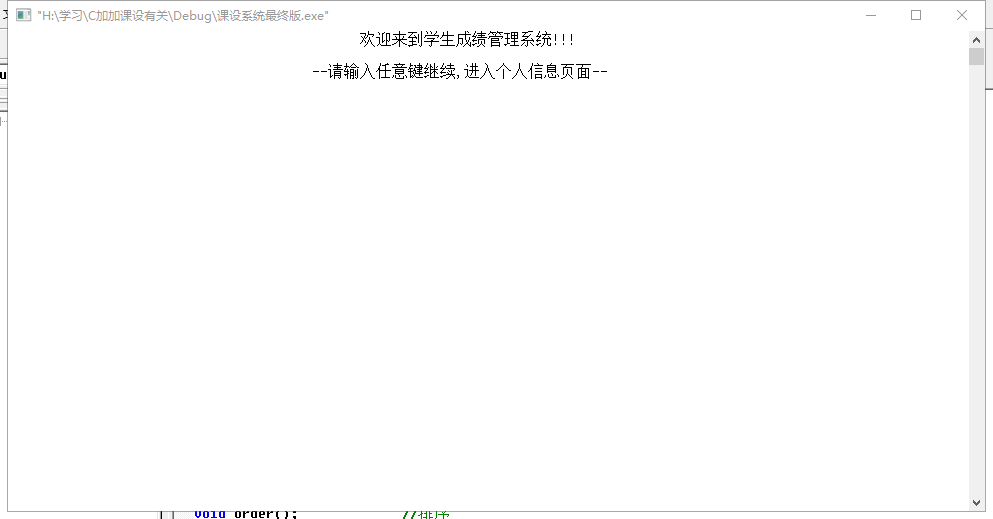首页先显示当前学生数据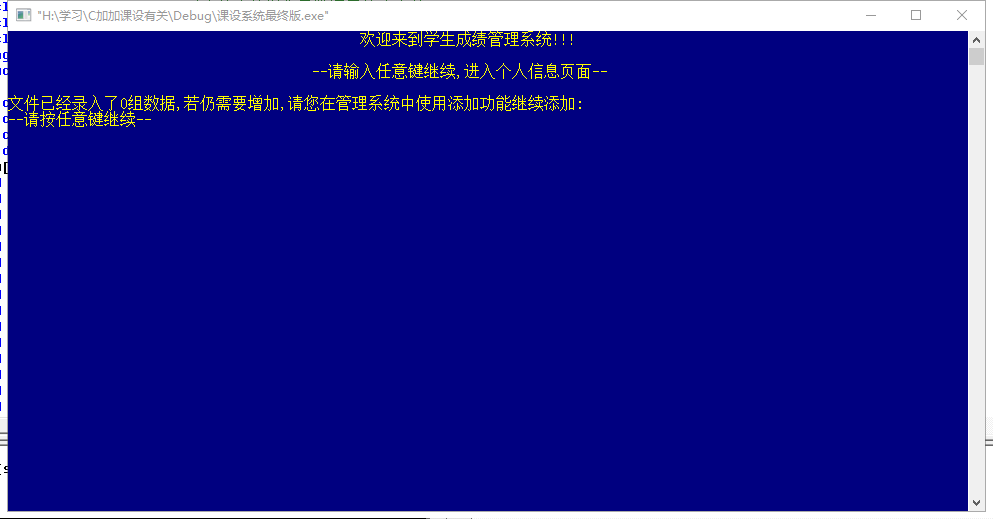程序功能选择页面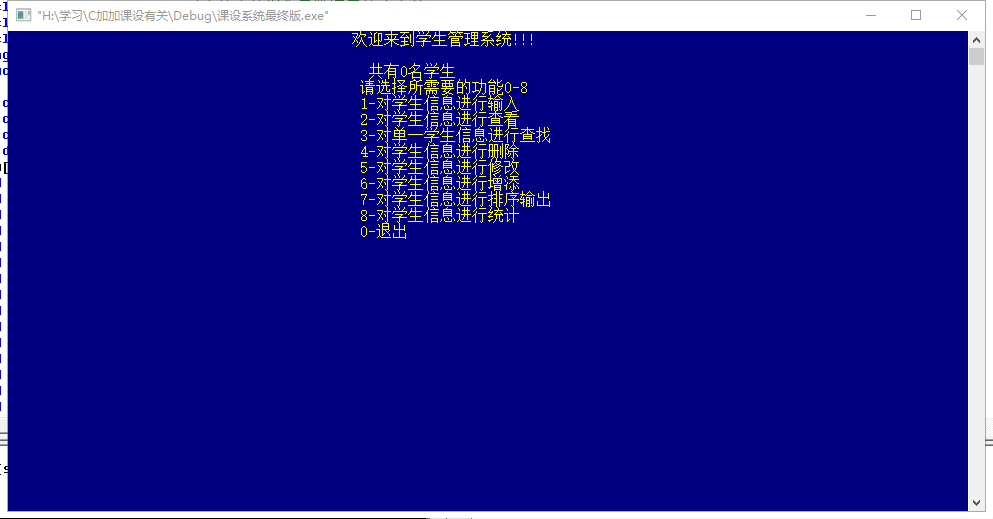这个程序是在刚学完c++的时候写的，现在看有很多不足之处，在学完Javaweb之后，我使用Javaweb又开发了一个学生信息管理系统，基于Javaweb的学生信息管理系统连接了数据库，功能也较为齐全可以供初学者参考。
Javaweb学生信息管理系统`
展开全文• 学生信息管理系统 对于C++这门课的课程设计的源代码
• 工具是Visual C++ 6.0，MFC开发的学生信息管理系统,设合做期末作业 和MFC入门学习案例
• 得安装myodbc驱动，创建数据库。 大家参考学习...

# c++写学生信息管理系统c++ 订阅